### Home > CALC > Chapter 7 > Lesson 7.1.3 > Problem7-31

7-31.
1. Consider the curve xe5y = 3y. Homework Help ✎

1. Find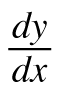.

2. Find the equation of the tangent line at (0, 0).

3. If x = 0.1, estimate y using the tangent line.

4. Using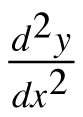, determine if the tangent approximation is an over or under estimate. Justify your answer in words.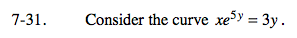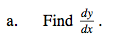Implicitly differentiate.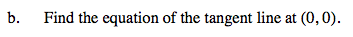Notice that the derivative you found in part (a) has both x- and y-values.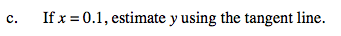Since x = 0.1 is easier to evaluate in a linear equation than in the curve shown above, use the tangent line to estimate the y-value at x = 0.1.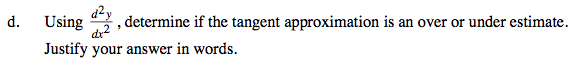$\text{When finding }\frac{d^{2}y}{dx^{2}}, \text{ remember to substitute your answer in part (a)}$

The sign of the 2nd derivative at (0,0) will determineif the tangent line is above or below the curve.

When a function is concave up, the tangent line will be below the curve.
When it is concave down, the tangent line will be above the curve.
Verify these statements by sketching a few examples.